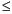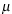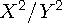Functions and CALL Routines

# FINV Function

Returns a quantile from the F distribution.
 Category: Quantile

## Syntax

 FINV (p, ndf, ddf <,nc>)

### Arguments

p

is a numeric probability.

 Range: 0p < 1
ndf

is a numeric numerator degrees of freedom parameter.

 Range: ndf > 0
ddf

is a numeric denominator degrees of freedom parameter.

 Range: ddf > 0
nc

is an optional numeric noncentrality parameter.

 Range: nc0

The FINV function returns the pth quantile from the F distribution with numerator degrees of freedom ndf, denominator degrees of freedom ddf, and noncentrality parameter nc. The probability that an observation from the F distribution is less than the quantile is p. This function accepts noninteger degrees of freedom parameters ndf and ddf.

If the optional parameter nc is not specified or has the value 0, the quantile from the central F distribution is returned. The noncentrality parameter nc is defined such that if X and Y are normal random variables with meansand 0, respectively, and variance 1, thenhas a noncentral F distribution with nc =2.

CAUTION:

For large values of nc, the algorithm could fail. In that case, a missing value is returned.Note:   FINV is the inverse of the PROBF function.These statements compute the 95th quantile value of a central F distribution with 2 and 10 degrees of freedom and a noncentral F distribution with 2 and 10.3 degrees of freedom and a noncentrality parameter equal to 2:

SAS Statements Results
`q1=finv(.95,2,10);`
`4.1028210151`
`q2=finv(.95,2,10.3,2);`
`7.583766024`

 Functions:Previous Page | Next Page | Top of Page